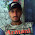# Adjustable Power Supply Using by LM317

Adjustable Power Supply Circuit Diagram

For the LM317:
• R2 = (192 x Vout) - 240, where R2 in ohms, Vout is in volts and must be at between 1.2 V and 35 V.
• Vin should be at least 2.5V greater than Vout. Select a wall adapter with a voltage at least 2.5 V greater than your regulated output at full load
•  Maximum output current is 1 A. Use proper heatsink for LM317 if it has to dissipate more than 1W.
• The tab of the LM317 is connected to the center pin.
Equation for calculating the output voltage when R1 and R2 are known:

Vout = 1.25V * (1 + R2/R1) + Iadj * R2

Where Iadj is typically in the range of 50 microamperes.

Share this article

#### 1 comments:

1.Thanks, Good explanation. Keep it up. :)